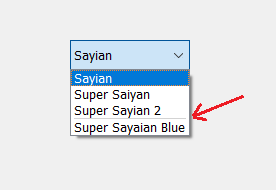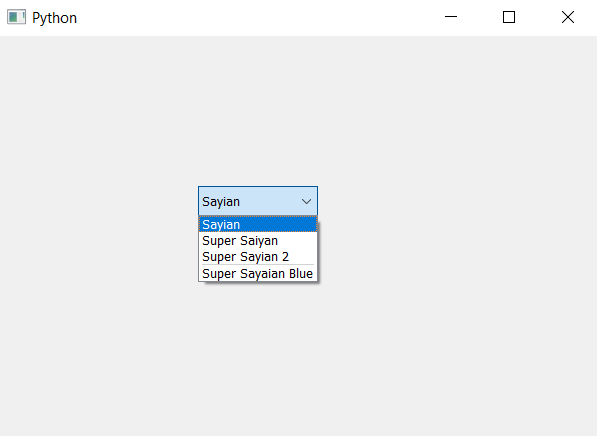# PyQt5 – How to add separator in between the item of ComboBox

• Last Updated : 22 Apr, 2020

In this article we will see how we can add separator at the desired index in the combo box. By default there is no separator. Separator is the black line in between two items, in order to add he separator we use `insertSeparator` method. Below is representation of combo box items with a separator looks like –Syntax : combo_box.insertSeparator(index)

Argument : It takes integer as argument i.e index

Action performed : It will add separator at the given index

Note: If the index is equal to or higher than the total number of items, the new item is appended to the list of existing items. If the index is zero or negative, the new item is prepended to the list of existing items.

Below is the implementation –

 `# importing libraries``from` `PyQt5.QtWidgets ``import` `*` `from` `PyQt5 ``import` `QtCore, QtGui``from` `PyQt5.QtGui ``import` `*` `from` `PyQt5.QtCore ``import` `*` `import` `sys`` ` ` ` `class` `Window(QMainWindow):`` ` `    ``def` `__init__(``self``):``        ``super``().__init__()`` ` `        ``# setting title``        ``self``.setWindowTitle(``"Python "``)`` ` `        ``# setting geometry``        ``self``.setGeometry(``100``, ``100``, ``600``, ``400``)`` ` `        ``# calling method``        ``self``.UiComponents()`` ` `        ``# showing all the widgets``        ``self``.show()`` ` `    ``# method for widgets``    ``def` `UiComponents(``self``):`` ` `        ``# creating a combo box widget``        ``self``.combo_box ``=` `QComboBox(``self``)`` ` `        ``# setting geometry of combo box``        ``self``.combo_box.setGeometry(``200``, ``150``, ``120``, ``30``)`` ` `        ``# geek list``        ``geek_list ``=` `[``"Sayian"``, ``"Super Saiyan"``, ``"Super Sayian 2"``, ``"Super Sayaian Blue"``]`` ` `        ``# adding list of items to combo box``        ``self``.combo_box.addItems(geek_list)`` ` `        ``# index``        ``index ``=` `3`` ` `        ``# adding separator at given index``        ``self``.combo_box.insertSeparator(index)`` ` ` ` `# create pyqt5 app``App ``=` `QApplication(sys.argv)`` ` `# create the instance of our Window``window ``=` `Window()`` ` `# start the app``sys.exit(App.``exec``())`

Output :My Personal Notes arrow_drop_up